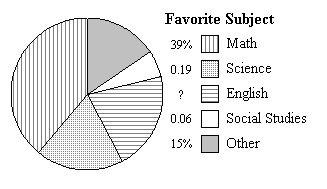Name:    Quiz 4.1 - 4.3

Multiple Choice
Identify the choice that best completes the statement or answers the question.

Write the percent as a fraction or mixed number in simplest form.

1.

2.5%
 a.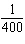c.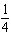b.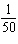d.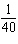2.

0.7%
 a.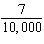c.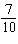b.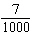d.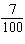Write the fraction as a percent.

3.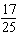a. 73% c. 66% b. 68% d. 64%

4.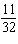a. 40.625% c. 34.375% b. 32.375% d. 37.375%

5.

So far, 22% of the flights you make with your remote control robotic flying toy stay in the air for longer than one minute. What fraction of flights is this?
 a.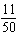c.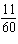b.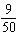d.Write the mixed number as a percent.

6.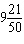a. 941% c. 946% b. 942% d. 938%

Write the percent as a decimal.

7.

4%
 a. 0.4 c. 0.04 b. 0.004 d. 4

8.

11.9%
 a. 0.119 c. 11.9 b. 0.0119 d. 1.19

Write the decimal as a percent.

9.

0.0353
 a. 0.0353% c. 35.3% b. 0.353% d. 3.53%

Tell which number is greater.

10.

0.014, 14%
 a. They are equal. b. 14% c. 0.014

11.

670%, 0.67
 a. 0.67 b. They are equal. c. 670%

Use a number line to order the numbers from least to greatest.

12.

30%,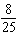, 0.31
 a. 0.31,, 30% c., 0.31, 30% b. 30%,, 0.31 d. 30%, 0.31,Order the numbers from least to greatest.

13.

66.666%,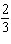, 0.667, 0.66
 a., 0.66, 66.666%, 0.667, c. 0.66, 66.666%, 0.667,, b. 0.66,, 66.666%, 0.667 d. 0.66, 66.666%,, 0.667

Numeric Response

Write the mixed number as a percent.

1.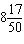2.

About 0.47 of the animals in a pet store were dogs. Write this decimal as a percent.

1.

Write three different proper fractions that are greater than 78%.

2.

Complete the table.

 Fraction Decimal Percent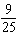0.36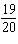95%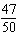0.2 70%

Other

1.

Extended Response  The percent of a wall covered by the mural painted by each grade is shown.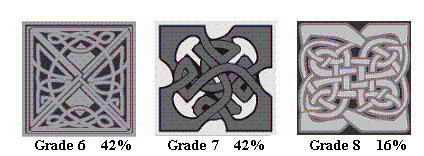a.   Write the percents as fractions in simplest form.